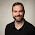Monday, March 19, 2012

How Fish Shoal (1): Understanding Regression

In late 2011 our research group at Uppsala University and our colleagues at the University of Sydney, published a study that aimed to show how fish in shoals change their speed and direction of movement in response to the other fish. We had placed groups of fish together in a tank and tracked their movements (see video below). How do we get from that to understanding how they respond to each other?

Let us begin by asking what we mean by saying 'we understand' why a fish changes its motion. My definition is that I understand why a fish does this if I can predict it. That is, I can say what a fish will do next if I know what its environment is like (I'm leaving the exact definition of 'the environment' intentionally vague at this point).

Environment → Next Behaviour

Mathematically we call this finding a mapping between the things on the left and the things on the right. We often write a mapping as a function, f.

Next Behaviour = f(Environment)

Understanding requires us to find how the environment influences behaviour. That is, we must find out what the function, f, is.

Having defined our goal, our next task is to make things a little more concrete. What behaviours are we interested in predicting? Which aspects of the environment do we think could be important? We chose to try to predict how a fish will change its speed and direction, using the positions and directions of the other fish around it. Let us consider only the change of speed, we now want to find a function such that:

speed change = f(positions of other fish, directions of other fish)

Now these are things we can actually measure from the recorded tracks (see figure below). So we can say where, for example, the nearest fish to our focal fish was at each moment, and correspondingly how much the focal fish accelerated or decelerated at that moment.

Measuring the position of the nearest fish

So have data giving both the inputs to the function (the positions/directions of the other fish), and the output (the acceleration of the focal fish). How do we learn what the function is. This task is generally known as regression. You will almost certainly have come across linear regression at school, which is a special case of this method, but generally regression simply means learning how one variable predicts another. Specifically we use the term 'regression' in contrast to 'classification': regression is used when we are dealing with a output (such as the acceleration) that can take many values. Classification is used for either/or type outputs, such a whether someone recovers from a disease.

In the next post we'll see how we can use our data to learn what the function mapping the environment to behaviour looks like, starting by recapping on the principles of linear regression...

1.Great work. Do you know where I can find more information on it?

1.The original study is published here: http://www.pnas.org/content/108/46/18726
Alternatively the next few blog posts give more details

2.Your blog is very useful for me.I really like you post.Thanks for sharing.

goldenslot
gclub
gclub casino

3.Hey guys I just want to say that I got an official notification that iTunes is providing us free gift cards codes iTunes Gift Card Codes